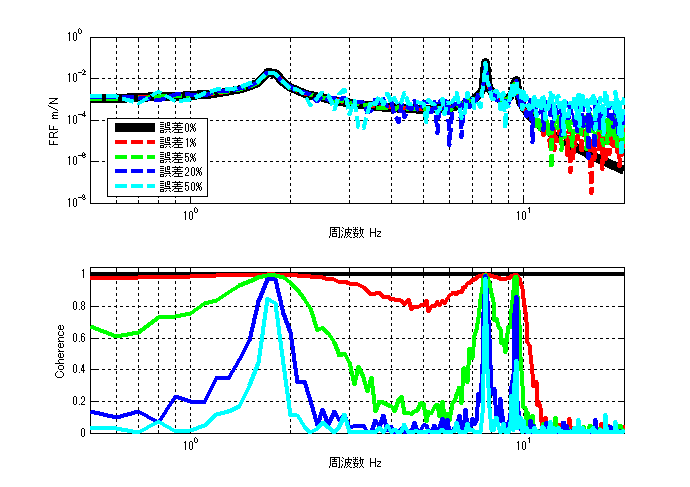# Coherence function of FRF

## Introduction

In the past, I have introduced several types of transfer functions (Frequency Response Function (FRF)): H1 estimation, H2 estimation, H3 estimation, and Hv estimation.

This article describes the FRF coherence function. The coherence is an indicator of how reliable the FRF is.

If you want to see all my lessons in English, please see below.

TopPage 振動騒音 研究所
MATLABとNVH(Noise, Vibration and Harshness)をこよなく愛する人生の先輩、それが私（MATLABパイセン）です。NVHを極めるにあたり、周辺の知識も網羅的に勉強することになり、その知識を共有すべく、本HPを運営しています。日本の製造業を応援すべく、機械エンジニアの「車輪の再開発」の防止と業務効率化の手助けをモットーに活動。専門である振動騒音工学や音響工学について、プログラムを示しながら解説。大学の授業よりもわかりやすく説明することを目指す。質問頂ければ、回答したいと思います。

## Coherence function

Some of you who are or have been studying vibration engineering may have tried to program a coherence function and have not been successful.

There are two complicated aspects of coherence functions.

#### 1st point:Why it’s complicated to understand

The first complication is that there are numerous formulas for coherence functions in textbooks and reference books. (However, they are the same when expanded? Shouldn’t they?)

For example, in “Introduction to Modal Analysis,” the coherence function is expressed in five equations. (The figure below is for reference.)

スポンサーリンク

$$γ^2_{fx}= \frac{W_{vv}}{W_{xx}}=\frac{\bar{V}V}{W_{xx}}=\frac{\bar{F H1}F H1}{W_{xx}}=\frac{\bar{H1}FF H1}{W_{xx}}=\frac{\bar{H1}W_{ff} H1}{W_{xx}}$$

$$γ^2_{fx}= \frac{(\bar{W_{fx}} / \bar{W_{ff}})W_{ff}(W_{fx} / W_{ff})}{W_{xx}}=\frac{\bar{W_{fx}} W_{ff}W_{fx}}{W_{xx}\bar{W_{ff}} W_{ff}}$$

$$γ^2_{fx}= \frac{\bar{W_{fx}}W_{fx}}{W_{ff}W_{xx}}= \frac{<W_{fx}W_{fx}>}{W_{ff}W_{xx}}$$

$$γ^2_{fx}= \frac{\|W_{fx}\|^2}{\|F\|^2\|X\|^2}$$

$$γ^2_{fx}= \frac{\|W_{fx}\|^2}{\|F\|^2\|X\|^2}$$

$$γ^2_{fx}(ω)= \frac{H1}{H2}$$

スポンサーリンク

#### 2nd point:Why it’s complicated to understand

The second complication is that it is unclear where the averaging should be done.

For example, in the equation below, it is unclear whether Wfx, etc., should be averaged by itself, or whether each denominator and numerator should be averaged, or whether the following equation should be obtained for the number of averaging times and then the averaging process should be performed.

$$γ^2_{fx}=\frac{\bar{W_{fx}} W_{ff}W_{fx}}{W_{xx}\bar{W_{ff}} W_{ff}}$$

#### Recommendation

In the equation below, the coherence function can be easily obtained as long as H1 and H2 are well obtained.

$$γ^2_{fx}(ω)= \frac{H1}{H2}$$

If you do not know the H1 or H2 estimates, please refer to the following.

## Validation in MATLAB

#### Analysis Results

The results of the analysis are shown first. (100 times average)With no error, the coherence function is 1, and as the error increases, the coherence function approaches 0.

Also, since the response is higher at resonant frequencies, the signal-to-noise ratio (signal-to-noise ratio) improves, which means that the coherence function is closer to 1.

#### MATLAB Code

###### Executable file
clear all
close all
% % % % 初期設定
freq=0.5:0.1:20;
m_vec=[1 1 1 1];
[M]=eval_Mmatrix(m_vec);
k_vec=[1 1 1 1]*10^3;
[K]=eval_Kmatrix(k_vec);
C=zeros(length(k_vec));
F=zeros(length(m_vec),length(freq));
F(1,:)=ones(1,length(freq));

% % % % 変位X（ノイズなし）
X=eval_direct_x_2ndedition(M,K,C,F,freq);
% % % % 理想的なFRF
FRF_ideal_x3_f1=X(3,:)./F(1,:);

N=100; %平均化回数 100回
res=zeros(N,length(freq));
res2=zeros(N,length(freq));
res3=zeros(N,length(freq));
res4=zeros(N,length(freq));
ref=zeros(N,length(freq));
for ii1=1:N
res(ii1,:)=X(3,:)+( rand(1,length(freq)) - 0.5 )*max(abs(X(3,:)))*0.01; % 最大変位Xを基準として1%のノイズを加える
res2(ii1,:)=X(3,:)+( rand(1,length(freq)) - 0.5 )*max(abs(X(3,:)))*0.05;
res3(ii1,:)=X(3,:)+( rand(1,length(freq)) - 0.5 )*max(abs(X(3,:)))*0.20;
res4(ii1,:)=X(3,:)+( rand(1,length(freq)) - 0.5 )*max(abs(X(3,:)))*0.50;
ref(ii1,:)=F(1,:);
end

[h1_1] = FRF(ref,res,freq,'H1');
[h1_2] = FRF(ref,res2,freq,'H1');
[h1_3] = FRF(ref,res3,freq,'H1');
[h1_4] = FRF(ref,res4,freq,'H1');
[r2_1] = Coherence(ref,res,freq);
[r2_2] = Coherence(ref,res2,freq);
[r2_3] = Coherence(ref,res3,freq);
[r2_4] = Coherence(ref,res4,freq);
[r2_0] = Coherence(ref(1,:),X(3,:),freq);

figure(1)
subplot(211)
loglog(freq,abs(FRF_ideal_x3_f1),'k','linewidth',7)
hold on
loglog(freq,abs(h1_1),'r--','linewidth',3)
loglog(freq,abs(h1_2),'g--','linewidth',3)
loglog(freq,abs(h1_3),'b--','linewidth',3)
loglog(freq,abs(h1_4),'c--','linewidth',3)
hold off
grid on
legend('誤差0%','誤差1%','誤差5%','誤差20%','誤差50%')
xlim([freq(1) freq(end)])
xlabel('周波数 Hz');ylabel('FRF m/N');

subplot(212)
semilogx(freq,r2_0,'k','linewidth',3)
hold on
semilogx(freq,r2_1,'r','linewidth',3)
semilogx(freq,r2_2,'g','linewidth',3)
semilogx(freq,r2_3,'b','linewidth',3)
semilogx(freq,r2_4,'c','linewidth',3)

xlabel('周波数 Hz');ylabel('Coherence');
grid on
ylim([0 1.05])
xlim([freq(1) freq(end)])


###### function file
function [frf] = FRF(ref,res,freq,FRFtype)

switch FRFtype
case 'H1'
[frf] = H1(ref,res,freq);
case 'H2'
[frf] = H2(ref,res,freq);
case 'H3'
[frf] = H3(ref,res,freq);
case 'Hv'
[frf] = Hv(ref,res,freq);
end

end

function [h1] = H1(ref,res,freq)

% h1=zeros(1,freq);

[Wfx]=CrossSpectralDensity(ref,res,freq);
[Wff]=PowerSpectralDensity(ref,freq);

h1=mean(Wfx,1)./mean(Wff,1);

end

function [h2] = H2(ref,res,freq)

% h1=zeros(1,freq);

[Wxf]=CrossSpectralDensity(res,ref,freq);
[Wxx]=PowerSpectralDensity(res,freq);

h2=mean(Wxx,1)./mean(Wxf,1);

end

function [h3] = H3(ref,res,freq)

[h1] = H1(ref,res,freq);
[h2] = H2(ref,res,freq);

h3 = (h1 + h2)/2;
end

function [hv] = Hv(ref,res,freq)

[h1] = H1(ref,res,freq);
[h2] = H2(ref,res,freq);

hv = sqrt(h1).*sqrt(h2);
end

function [r2] = Coherence(ref,res,freq)

[h1] = H1(ref,res,freq);
[h2] = H2(ref,res,freq);

r2=h1./h2;

end

function [Wff]=PowerSpectralDensity(F,freq)

if size(F,1)==length(freq)
F=F.';
end

Wff=conj(F).*F;
end

function [Wfx]=CrossSpectralDensity(F,X,freq)

if size(F,1)==length(freq)
F=F.';
end
if size(X,1)==length(freq)
X=X.';
end

Wfx=conj(F).*X;
end

function [K]=eval_Kmatrix(k_vec)
K=zeros(length(k_vec));
for ii1=1:length(k_vec)
if ii1==1 K(ii1,ii1)=k_vec(ii1);
else
K(ii1-1:ii1,ii1-1:ii1)=K(ii1-1:ii1,ii1-1:ii1)+[1 -1;-1 1]*k_vec(ii1);
end
end

end

function [M]=eval_Mmatrix(m_vec)
M=diag(m_vec);
end

function x=eval_direct_x_2ndedition(M,K,C,F,freq)
% % 2021.05.28 Fを修正
x=zeros(size(F,1),length(freq));
j=sqrt(-1);
for ii1=1:1:length(freq)
w=2*pi*freq(ii1);
x(:,ii1)=inv(K+j*w*C-w^2*M)*F(:,ii1);
end

endMATLABパイセンが教える振動・騒音・音響・機械工学